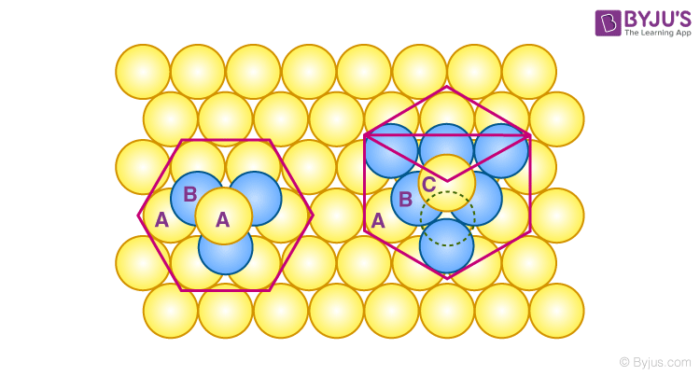Checkout JEE MAINS 2022 Question Paper Analysis : Checkout JEE MAINS 2022 Question Paper Analysis :

# Close Packing In Solids In Three Dimensions

Crystalline solids exhibit a regular and repeating pattern of constituent particles. The diagrammatic representation of three-dimensional arrangements of constituent particles in a crystal, where each particle is depicted as a point in space is known as a crystal lattice. In a crystal lattice, the atoms are very closely packed, leaving very little space between them. Close packing in solids in three dimensions is discussed below:• #### Three-dimensional close packing from two-dimensional square close-packed layers:

In this kind of close solid packing, the second layer is placed over the first layer in such a way that the spheres of the upper layer are exactly above those of the first layer. In other words, spheres of both the layers are perfectly aligned, horizontally and vertically. Let us name the arrangement of spheres in the first layer as ‘A’ type since all the layers have the same arrangement, the lattice can be observed to follow AAA…. type pattern. This kind of lattice is better known as a simple cubic lattice.

• #### Three-dimensional close packing from two-dimensional hexagonal close-packed layers:

The three-dimensional close-packed structure can be generated by placing layers one over the other.

#### Placing the second layer over the first layer:

In this kind of close packing, a second layer similar to the below layer is placed in such a way that the spheres of the second layer are placed in the depressions of the first layer. Since the spheres of the two layers are aligned differently, if the first layer is termed as ‘A; the second layer can be termed as ‘B’. We notice that a tetrahedral void is formed wherever a sphere of the second layer is above the void of the first layer (or vice versa). Whereas at other places, we observe that the triangular voids in the second layer are above the triangular voids in the first layer, such that the triangular shapes of these do not overlap. Such voids are known octahedral voids and are surrounded by six spheres.

We can easily calculate the number of these two types of voids. Let the number of close packed spheres be N, then:

The number of octahedral voids generated = N

The number of tetrahedral voids generated = 2N

#### Placing the third layer over the second layer:

There are two prominent ways in which the third layer can be placed over the second layer:

## Covering Tetrahedral Voids

In this kind of close packing in solids, the spheres of the third layer are exactly aligned with those of the first layer. Thus, if we name the first layer as ‘A’ and second as ‘B’, then the third layer can again be named as ‘A’. This pattern can be written as ABAB ……. pattern. This structure is better known as a hexagonal close-packed (hcp) structure.

## Covering Octahedral Voids

In this kind of close packing, the spheres of the third layer are not aligned with those of either the first or the second layer. Thus, if we name the first layer as ‘A’ and second as ‘B’, then the third layer will be named as ‘C’. This pattern of layers can be written as ABCABC……….. This crystal structure is called cubic close-packed (ccp) or face-centered cubic (fcc) structure.

In both of them, the coordination number is 12 as each sphere is in contact with twelve spheres.74% space in the crystal is filled in these kinds of close packing.

## Recommended Videos## Frequently Asked Questions – FAQs

### What is close packing in solids?

Near crystal packing refers to the effective arrangement of constituent particles in a crystal lattice in vacuum. We have to assume that all particles (atoms , molecules and ions) are of the same spherical solid form to grasp this set more precisely. So a cubic structure is the unit cell of a lattice.

### How many types of close packing are known in crystals?

Inside a unit cell, the most powerful conformation atomic spheres can take is known as the nearest packing configuration. In two modes, there are closely packed atomic spheres: hexagonal closest packing (HCP) and cubic closest packing (CCP).

### What is the difference between hexagonal close packing and cubic close packing?

The tight packing of the hexagon is denoted as HCP. In one repeated unit, this arrangement has two layers of spheres. The main distinction between hexagonal close packing and cubic close packing is that there are 6 spheres in a unit cell of hexagonal close packing, whereas there are 4 spheres in a unit cell of cubic close packing.

### What is hexagonal packing?

Hexagonal tightly packed (hcp) refers to layers of packed spheres such that spheres overlay each other in alternating layers. A slip framework, which is a close-packed structure, is hexagonal near packed. For elemental metals, the hcp structure is very general, including: beryllium.

### What is the packing efficiency?

The packing quality is the proportion of the atoms directly occupied by the crystal (or unit cell). It must often be less than 100 percent, since spheres (atoms are usually spherical) can not be filled without any empty space between them.

For a detailed discussion on close packing in three dimensions, download BYJU’S – The Learning App.

Test your Knowledge on Close packing three dimensions!